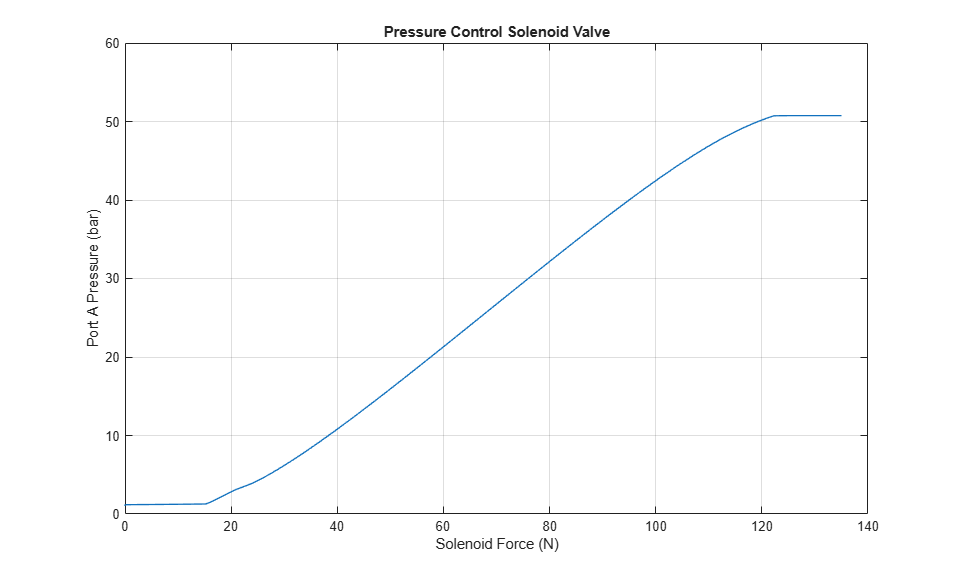# Pressure Control Solenoid

This example shows how to model, parameterize, and test a pressure control solenoid valve. This example also generates a plot of the relationship between applied solenoid force and the resulting actuator port pressure.

### Model

The following figure shows the model of a pressure control solenoid valve. Here P represents the pressure source port,T represents the reservoir, and A represents the actuator port.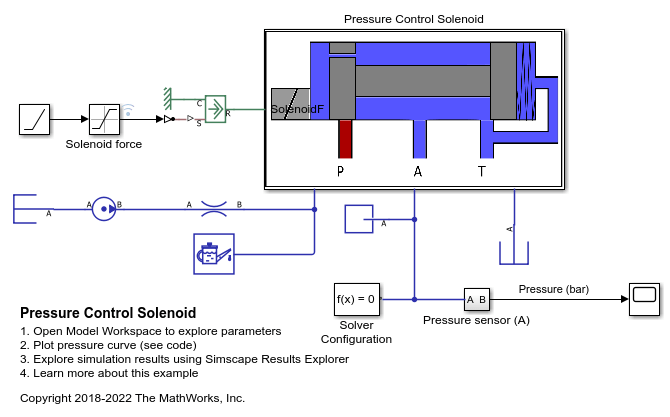### Pressure Control Solenoid Subsystem

This subsystem shows how the pressure control solenoid valve is modelled.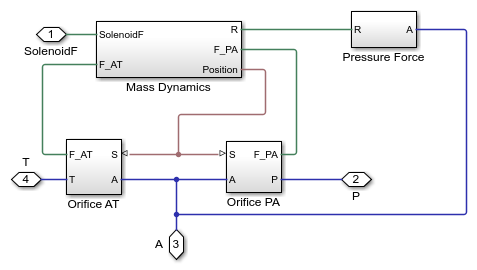### Mass Dynamics Subsystem

This subsystem shows how the spool mass dynamics are modelled, including the forces on the spool due to spool inertia; the forces on the spool due to the spring, damper, and hard stop; friction between the spool and the valve body; the change in fluid momentum at the valve entry and exit; and the pressure acting on the spool surface.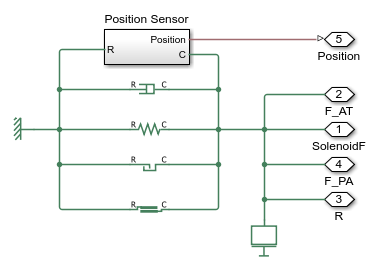### Pressure Force Subsystem

This subsystem shows how the fluid pressure at port A is modelled as a force on the spool left face.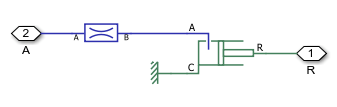### Orifice AT Subsystem

This subsystem shows how the orifice between ports A and T is modelled.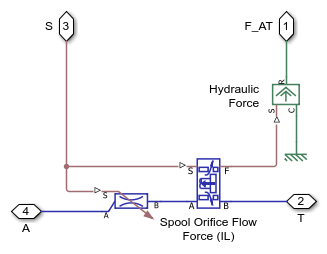### Orifice PA Subsystem

This subsystem shows how the orifice between ports A and P is modelled.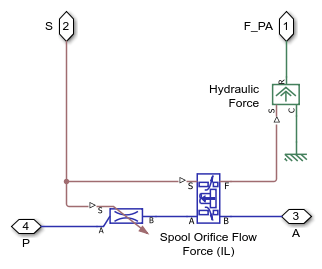### Simulation Results from Simscape™ Logging

This model generates a plot between the applied solenoid force and the generated actuator port pressure.